Continuing off of yesterday’s blog post of Love Bit(e)s, now with the basis of binary arithmetic it’s a lot easier to understand bitwise operations!

#### What is a Bitwise Operator, and why do I care?

A bitwise operation manipulates bits for calculations or comparisons. They are insanely fast and efficient.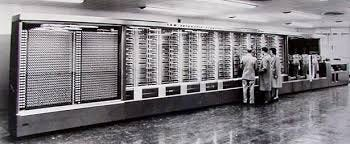Harvard Mark I

When the Harvard Mark I was built in 1944 it used bitwise operators AND, OR and XOR to do computations. Alan Turing, noted as the father of computers, when writing the manual for the Harvard Mark I elaborated on how a bitwise NOT could be gained.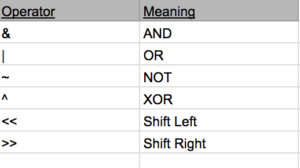These are the operators that will be covered, even though there are plenty more, we’ll keep it simple.

Remember to read all these operations go from right to left!

#### AND (&) Operator

The AND operator compares each binary digit of two integers and returns a new integer. The AND operator does this by multiplying them, so we could see the algebraic representation of this operator as:

``````Z = A * B
``````

The logic gate for AND would look something like: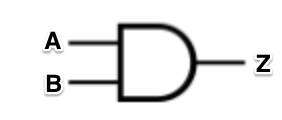To understand the AND operator, we could put it in action. Take for example the binary number 0101 which is equal to seven, and the binary number 0011, which is three.

``````0101AND 0011    0001
``````

Starting at the rightmost integer,

``````1 * 1 = 1 0 * 1 = 0 1 * 0 = 0 0 * 0 = 0

So, we ended up with 0001 which is equal to one. This can be typically useful in bit masking, where you want to see if only one bit is active. For example, if we wanted to clear all bits in 0101 (seven) except for the second bit, we could do so like:

0101AND 0100    0100

We can use the zeros like how masking tape while painting would be used, covering up the parts we don't want to see! We can also use the AND operator to check the parity (even or odd) of a number by checking it's lowest valued bit.

0101AND 0001    0001

Since 7 and 1 is 1, we know it is not divisible by two and therefore odd.

OR (|) Operator

The OR operator compares each binary digit across two integers and gives back a 1 if either of them have a 1. The algebraic expression for this looks like:

Z = A + B

The logic gate for OR looks like:
``````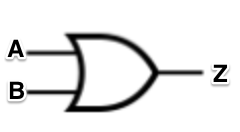``````We can take the same example above, but just change the operator:

0101OR 0100   0101

Starting at the rightmost integer:

1 + 0 = 10 + 0 = 01 + 1 = 1 0 + 0 = 0

The OR operator is very useful in finding which values are set to 1.

NOT (~) Operator

The NOT operator, or complement, gives the ones' complement of a binary integer. Bits that are 0 become 1, and bits that are 1 become 0.

The algebraic expression for this looks like (the sign above the A is the sign used to represent NOT):

Z = Ā

The logic gate for NOT looks like: (the little circle means the 1 and 0s should be flipped!):
``````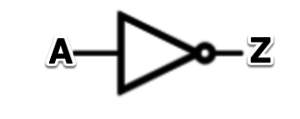``````If we were to take seven and try it with NOT, the output would look like:

NOT 0101    1010

The NOT operator could be used to help finding two's complement, which we talked about in the previous post.

XOR (^) Operator

The XOR operator takes takes two integers and if there is a 1 in both positions, it returns a 0, if there is only a single 1 in either position, it will return a 1, but if there is a 0 position in both positions it will return 0.

The algebraic expression for this would be:

Z = A
````````````B

The logic gate for XOR looks like:
``````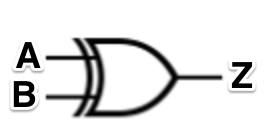``````But the logic gate for XOR is actually a combination of AND, OR, and NOT gates:
``````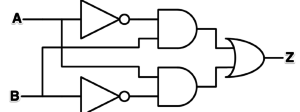``````If we preformed a XOR on two, we could do it like such:

0010

XOR 1010

1000

Preforming XOR against itself will always produce zero, which is how assembly programmers sometimes short-cutted and set the register to zero.

Bit Shifts

Bit shifts either move digits to the left or right. They are common due to the fact registers only have a fixed width, so some bits can be "shifted out" of the register in one end, while other bits are "shifted in" on the other. There are many types of shifts (arithmetic shifts, logical shifts, rotate no carry, etc) but for this post I'll only be covering arithmetic shifts and logical shifts.

Arithmetic Shifts

In an arithmetic shift the bits that are shifted out are discarded. In a left arithmetic shift, zeros are shifted in on the right.

A left shift would look like:

<< 0101 = 1010

In a right arithmetic shift, the sign bit (which is in the most significant bit position, or the left most side) is shifted in on the left (therefore it does not change the sign of the value)

Where a right shift would look like:

>> 0101 = 1101
``````

#### Logical Shifts

``````In a logical shift, zeros are shifted in to replace bits. A logical left shift and a logical arithmetic shift are the same.

<< 0101 = 1010

Logical shifts do not care about the sign of the number, so during a right logical shift 0s are just inserted. These are ideal for signed two's complement numbers.

>> 0101 = 0010

Conclusion

There are a lot of ways to change, manipulate, and calculate things in binary. The most amazing part is how insanely fast this occurs. Understanding how bits can be manipulated and calculated is like understanding atoms, and how they make up the world around us!

Here's a good video on everything

I used to think programming was lame and cheating, but now I just find it a useful way to avoid doing math.. except I keep ending up learning math anyways.
``````